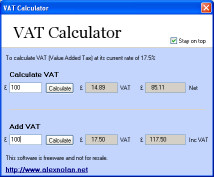## Calculate VAT

### How to calculate VAT

The calculator below will calculator the net amount from an amount that already has VAT included in it to give you the VAT and the net.

## To calculate VAT at 15%

 £   £VAT       £Net Amount

The calculator below will add the current VAT rate of 15% to a net amount.
Enter a value in pound sterling in the first box and click Calculate VAT.